## Electrical Calculator is a simple app to calculate Current in Amperes from Kilo Volt Amperes (KVA), Kilo Watts (KW) from Kilo Volt Amperes (KVA), Kilo Volt Amperes (KVA) from Kilo Watts (KW), HorsePower from Kilo Watts (KW), Kilo Volt Amperes (KVA) from HorsePower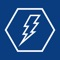# Electrical Calculator Mobile

by ITAMCO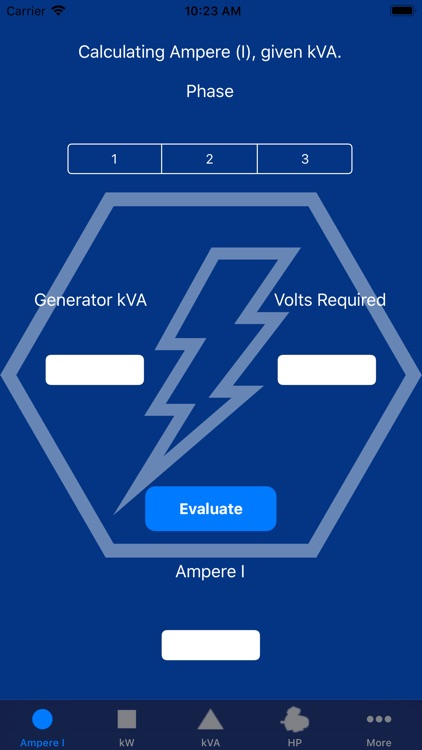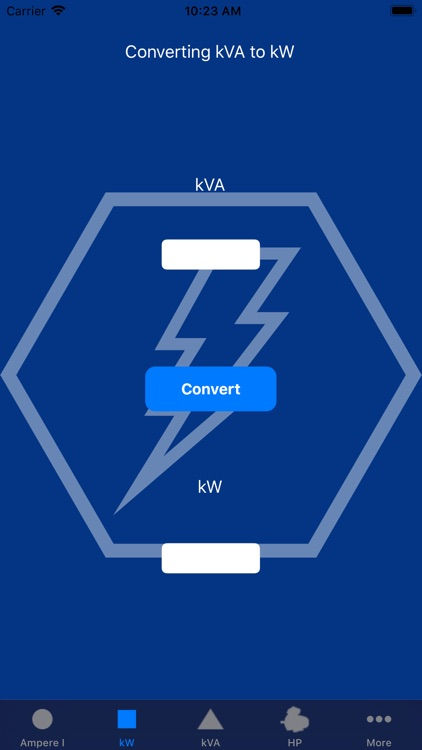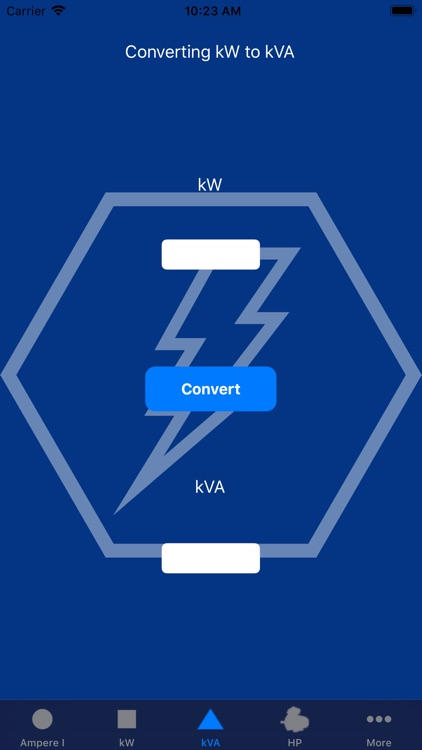Electrical Calculator is a simple app to calculate Current in Amperes from Kilo Volt Amperes (KVA), Kilo Watts (KW) from Kilo Volt Amperes (KVA), Kilo Volt Amperes (KVA) from Kilo Watts (KW), HorsePower from Kilo Watts (KW), Kilo Volt Amperes (KVA) from HorsePower. For reference use only.### App Details

Version
2.0
Rating
(1)
Size
14Mb
Genre
Productivity Utilities
Last updated
September 18, 2018
Release date
February 13, 2013

### App Screenshots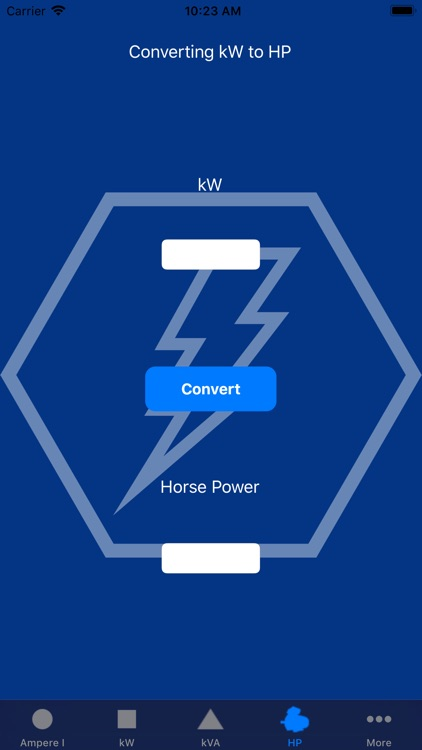### App Store Description

Electrical Calculator is a simple app to calculate Current in Amperes from Kilo Volt Amperes (KVA), Kilo Watts (KW) from Kilo Volt Amperes (KVA), Kilo Volt Amperes (KVA) from Kilo Watts (KW), HorsePower from Kilo Watts (KW), Kilo Volt Amperes (KVA) from HorsePower. For reference use only.